## Two Dimensional Motion in a Uniform Electric Field:

Two Dimensional Motion in a Uniform Electric Field – Let us consider that an electron enters the region between two parallel plates of a parallel plate capacitor oriented as illustrated in Fig. 3.5 with an initial velocity vox m/s in the +X direction. It is assumed that the electric field ε between the plates is uniform and, as chosen, it is in the direction of –Y axis, no other fields existing in the region.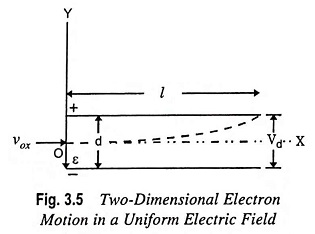The initial condi­tions, at t = 0, are as­sumed to be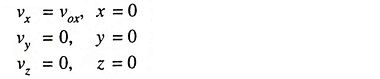Since there is no force in the Z direc­tion, the acceleration in that direction is zero and, therefore, the component of veloc­ity in the Z direction remains constant. Since the initial velocity in this direction is assumed to be zero, the motion must take place entirely in one plane, the plane of the paper or X-Y plane.

For a similar reason the velocity along the X-axis remains constant and is equal to vox i.e.,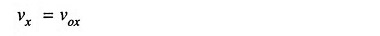It means that the distance moved in time t in X-direction is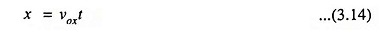On the other hand, a constant acceleration exists along the Y-direction, and the two dimensional motion in a Uniform Electric Field is given by equation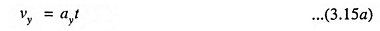and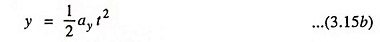wherewhere

• Vd is the potential applied across the plates and d is the separation.

The above equations indicate that in the region between the plates, the electron is accelerated upward, the velocity component vy varying from point to point, whereas the velocity component vx remains unchanged in the passage of the electron from one plate to another one. The path of movement of electron with respect to point O is readily known by combining Eqs. (3.14) and [3.15(b)], the variable t being eliminated.